# Study of the Factors Influencing Microstructure Transformation in the Billet Casting Process

Study of the Factors Influencing Microstructure Transformation in the Billet Casting Process

Shaowu Lei Jiongming ZhangXinkai Zhao Qipeng Dong

State Key Laboratory of Advanced Metallurgy, University of Science and Technology in Beijing, 100083, China

Corresponding Author Email:
lsw3601144@gmail.com
Page:
115-120
|
DOI:
http://doi.org/10.18280/ijht.330317
N/A
|
Accepted:
N/A
|
Published:
30 September 2015
| Citation

OPEN ACCESS

Abstract:

A slice numerical simulation model of transient heat transfer during the solidification process has been developed to study the effect of superheat, casting speed and cooling rate on the ratios of the columnar grain, equiaxed grain and mixture of the columnar grain and equiaxed grain in the billet casting process. The effect of each factor on the ratios of the columnar, equiaxed and mixture in the billet casting process is determined. The results show that the surperheat and casting speed have little effect on the ratio of the equiaxed area and that increasing surperheat and casting speed helps to increase  the ratio of the columnar, but it is not good for the increasing of ratio of the mixture. The cooling rate shows little effect on the ratios of the columnar, equiaxed and mixture.

Keywords:

Microstructure transfer, CET model, Slice model, Heat transfer.

1. Introduction

It is a common phenomenon that the solidification microstructure changes from an outer columnar to an inner equiaxed grain structure in the metal alloy casting process, and the mathematical model for the columnar to equiaxed transition (CET) has been proposed based on the experiment data . The CET model during alloy solidification  has had limited success due to the complex interplay of macroscopic phenomena, such as heat transfer and fluid flow, and microscopic phenomena such as nucleation and dendritic growth . Many works [1, 3-7] have studied the factors influencing the columnar to equiaxed transition, but there are few papers that studied factors influencing the ratio of the columnar, equiaxed and mixture in the steel casting process.  Understanding these three factors together is important for a steel company to improve the inner quality of continuous casting billet.

Cellular automata model is a kind of stochastic simulation method, Gandin, C. and Rappaz, M. [8, 9], Nastac, L. and Stefanescu, D. M. [10, 11] studied the Cellular automata model, and Gandin, C. and Rappaz, M. [8, 9] used the CAFE (Cellular Automata and Finite Element) model to study the CET of the melt. It can produce more information for the billet solidification, but it hits a bottleneck for simulation since it is difficult to compute and analyze statistically.

This work has studied the influencing factors on the columnar to equiaxed transition by using the CET model to determine the scope of the columnar, equiaxed and mixture and comparing with the calculated results of the CAFE model after computing the heat transfer and solidification of the billet by using the slice model for the billet casting

process. The work of this paper coincides with the calculating results  of the CAFE model.

2. Mathematical Model

2.1 Assumptions in this model

The following assumptions have been included in the present mathematical model for the complexity of the heat transfer phenomena during the solidification process of liquid steel.

(1) The molten steel is considered as a steady and incompressible Newton flow because the heat transfer model is a steady model.

(2) Without considering the fluctuation of molten steel in the mold, the top surface is covered with a protective slag layer which keeps the surface thermally insulated from the surroundings. The boundary condition of the top surface is a wall and the heat flux is zero.

(3) The caster is perfectly vertical with respect to the gravitational field and the curvature of the strand is ignored. Because the heat transfer model is a 2-D transient model, it cannot consider the effect of the curvature of the strand.

(4) Only the evolution of latent heat due to the phase of change from liquid to solid is taken into account. Because it's necessary for a reasonable simplified model.

(5) Physical parameters of the steel are constant, because it is necessary for a reasonable simplified model.

2.2 Heat transfer model

The temperature in the billet shell is governed by the 2-D transient heat-conduction equation, which becomes the following without considering flow and heat transfer in the casting direction:

$\frac{\partial}{\partial t}(\rho H)=\nabla \cdot(k \nabla T)$     (1)

Where $H$ is reference enthalpy; $\rho$ is liquid steel density; $k$ is thermal conductivity; $T$ is temperature.

The enthalpy of the material $H$ is computed as the sum of the sensible enthalpy h and the latent heat $\Delta H$:

$H=h+\Delta H$     (2)

$h=h_{r e f}+\int_{T_{r e f}}^{T} c_{p} d T$    (3)

$\Delta H=f \cdot H_{\text {latentHeat}}$    (4)

Where $h_{r e f}$ is reference enthalpy; $T_{r e f}$ is reference temperature; $c_{p}$ is specific heat at constant pressure; $f$ is liquid fraction.

The liquid fraction $f$ can be defined as:

$f=\left\{\begin{array}{l}0 \cdots\left(T<T_{\text {solidus}}\right) \\ \frac{T-T_{\text {solidus}}}{T_{\text {liquidus}}-T_{\text {solidus}}} \cdots\left(T_{\text {solidus}}<T<T_{\text {liquidus}}\right) \\ 1 \cdots\left(T>T_{\text {liquidus}}\right)\end{array}\right.$     (5)

The compute zone is a slice cutting from the billet, and its boundary is a function of the time t shown in Figure 1. The casting length (m), indicates that Q (t) or h (t) is the local boundary condition for the billet which is shown in section 2.4.

## 1.png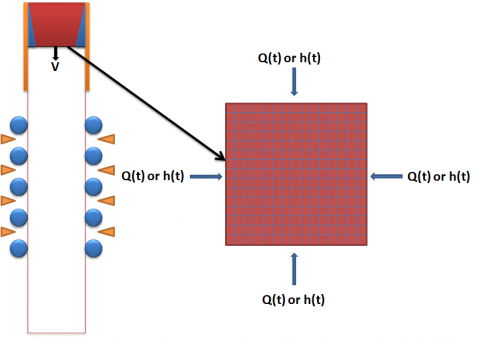Figure 1. Slice model for the billet casting process

Liquidus and solidus temperatures depend on steel com-position as follows :

$T_{l i q}=T_{p u r e}-\sum_{i} m_{i} C_{0, i}$     (6)

$T_{s o l}=T_{p u r e}-\sum_{i} m_{i} C_{L, i}$      (7)

According to the solute transport theory, the solute concentration in the liquid must be written as follows:

$C_{L}=C_{0} / k^{\gamma / L}$    (8)

The parameter for the liquidus and solidus temperatures are given in Table 1, where $C_{0}$ is the initial solute concentration, $k^{\gamma / L}$ is the partition coefficients for the process, m is a constant for the element.

Table 1. Parameter for the liquidus and solidus temperatures 

 Element C0(%) kγ/L m(℃/%) C 0.7 0.34 78 Si 0.2 0.52 7.6 Mn 0.65 0.78 4.9 P 0.011 0.13 34.4 S 0.0023 0.035 38

2.3 CET model

The CET model was developed by Hunt in 1984, the form $\nabla T \sim\left(C_{0} V\right)^{0.5}$ was used to describe the relationship of the undercooling with the alloy composition and growth rate. After considering the relationship $d \nabla T / d t=-V G$ , the radius of the equiaxed grains after time t was defined by the following equation:

$r=\int_{\Omega_{n}}^{\Delta T_{c}}\left(\frac{K \Delta T^{2}}{V G C_{0}}\right) d(\Delta T)=\frac{K\left(\Delta T_{c}^{3}-\Delta T_{n}^{3}\right)}{2 V G C_{0}}$    (9)

Where $\Delta T_{c}$ is the undercooling at the front of columnar growth; $\Delta T_{n}$ is the undercooling at the heterogeneous nucleation temperature; V is the solidification velocity; G is the temperature gradient;  $C_{0}$ is the alloy composition.

The fully equiaxed growth occurs when the extended volume fraction $\phi_{E}>0.66$ , and the full columnar growth occurs when the extended volume fraction $\phi_{E}<0.0066$  according to the theory of Hunt in 1984,$\phi_{E}$ is defined by the following equation:

$\phi_{E}=\frac{4 \pi r^{3} N_{0}}{3}$    (10)

The final result for the columnar-equiaxed transition for the specific steel is:

$G<0.6 N_{0}^{1 / 3} \Delta T_{c}\left(1-\frac{\Delta T_{n}^{3}}{\Delta T_{c}^{3}}\right)$ :fully equiaxed   (11)

$G>2.9 N_{0}^{1 / 3} \Delta T_{c}\left(1-\frac{\Delta T_{n}^{3}}{\Delta T_{c}^{3}}\right)$   :fully columnar   (12)

After considering $\frac{\Delta T_{n}}{\Delta T_{c}}<<1$ and $\Delta T_{c}=\left(V C_{0} / A\right)^{0.5}$ , the criterion of the columnar to equiaxed transition can be simplified as:

$\frac{G}{V^{0.5}}<0.6 N_{0}^{1 / 3}\left(\frac{C_{0}}{A}\right)^{0.5}$   :fully equiaxed      (13)

$\frac{G}{V^{0.5}}>2.9 N_{0}^{1 / 3}\left(\frac{C_{0}}{A}\right)^{0.5}$  :fully columnar     (14)

Where A is a constant; $N_{0}$ is the total number of heterogeneous substrate particles originally available per unit volume.

After considering A, $N_{0}$ are constants for the specific steel, then Eq. (13) and Eq. (14) can be further simplified as:

$\frac{G}{V^{0.5}}<0.2 C_{s t}$ :fully equiaxed    (15)

$\frac{G}{V^{0.5}}<C_{s t}$  :fully columnar    (16)

$C_{s t}=2.9 N_{0}^{1 / 3}\left(\frac{C_{0}}{A}\right)^{0.5}$     (17)

Then $C_{s t}$ can be obtained from the comparison of this work with the experimental result. The experimental result and the result calculated by CET model are shown in Figure 2, under conditions of 25K surperheat and 0.03m/s casting speed. The concluding value of about 500000 can be obtained from the comparison. So $C_{s t}=500000$ is used as the criterion for the CET model.

## 2.pngFigure 2. a) The experimental result;

b) Result calculated by CET model;

c) Comparison of the experimental result with the result calculated by the CET model.

2.4 Material properties and boundary condition

The material properties are defined as below:

Table 2. Properties of the steel and boundary condition

 Parameters Values Dimensions $C_{p}$, Specific Heat 700 J/kg/K k, Thermal Conductivity 31 W/m/K ρ, Density 7000 $k g / m^{3}$ $H_{\text {LatentHeat}}$ , Latent Heat 264000 J/kg $U_{c a s t}$ , Casting speed 0.017, 0.023, 0.030, 0.037, 0.043 m/s $\Delta T_{s}$ , Surperheat 5, 15, 25, 35, 45 K $T_{f}$ , Ambient temperature 25 K $T_{\text {pure}}$ , Melting point of pure iron 1809 K A, Solidification constant 3e-4 M %/s/K2

The expression determined empirically by Davies et al.  based on the data gathered at the Port Kembla Works of BHP is used for mould heat flux, the expression is defined as follows:

$Q=2.64 \cdot \exp \left(\frac{-z}{U_{\text {cast}}}\right)+0.91 \cdot \exp \left(\frac{-z}{11.3 U_{\text {cast}}}\right)+0.93$     (18)

Where Q is the heat extraction rate in megawatts per square meter; z is the distance below the meniscus in meters; $U_{c a s t}$ is the casting speed in meters per second.

The secondary cooling conditions are described as follows:

$\frac{\partial H}{\partial x}=\frac{\partial H}{\partial y}=-\frac{\gamma}{k}\left(H_{s}-H_{a}\right)$    (19)

Where γ is the average heat transfer coefficient between the solid surface and the surrounding; $H_{s}$ represents the enthalpy at the surface; $H_{a}$ represents the product of ambient temperature and the specific heat of steel.

Table 3. Parameters for the boundary condition

 Zone mold roller 1 2 3 Air length/m 0.9 0.37 2.6 4.2 2.2 10 average heat transfer coefficient $\gamma$/ W.m-2.K-1 - 434 240 178 152 20
3. Results and Discussion

The slice model has been developed to study the solidification in the billet caster, the slice is 0.17m$\times$0.17m which element size is 0.001m, calculated length is 15m. The effects of surperheat, casting speed and cooling rate on the ratio of the columnar, equiaxed and mixture are studied in the following paper.

The area of the columnar, equiaxed and mixture can be by using the criterion of the columnar to equiaxed transition, then the ratio $\varphi_{\text {columnar}}$ , $\varphi_{\text {equiaxed}}$ and $\varphi_{\text {mixture}}$ can be obtained from the following equations:

$\varphi_{\text {columnar}}=\frac{S_{\text {columnar}}}{S_{\text {columnar}}+S_{\text {equiaxed}}+S_{\text {mixture}}}$    (20)

$\varphi_{\text {equiaxed}}=\frac{S_{\text {equiaxed}}}{S_{\text {columnar}}+S_{\text {equiaxed}}+S_{\text {mixture}}}$    (21)

$\varphi_{\text {mixture}}=\frac{S_{\text {mixture}}}{S_{\text {columnar}}+S_{\text {equiared}}+S_{\text {mixture}}}$    (22)

Where $S_{\text {columnar}}$ is the area of the columnar; $S_{\text {equiaxed}}$ is the area of the equiaxed; $S_{\text {mixture }}$ is the area of the mixture.

3.2 Effects of surperheat on the ratio of the columnar, equiaxed and mixture

The results calculated by CAFE model  are shown in Figure 3, and the predictions of the CET model are shown in Figure .

## 3.png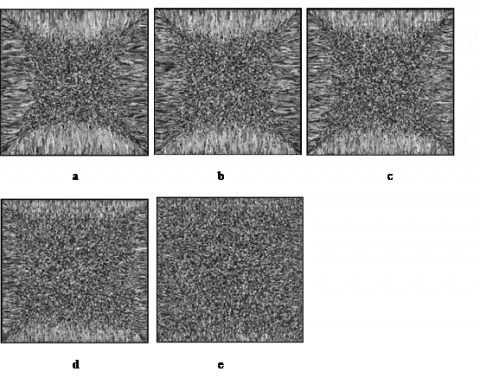Figure 3. Calculated by CAFE model  with $U_{\text {cast}}$=0.03m/s a) $\Delta T_{s}$=45K b) $\Delta T_{s}$=35K c)$\Delta T_{s}$=25K d)$\Delta T_{s}$=15K e)$\Delta T_{s}$=5K

## 4.pngFigure 4. Calculated by CET model with $U_{c a s t}$=0.03m/s a) $\Delta T_{s}$=45K b) $\Delta T_{s}$=35K c)$\Delta T_{s}$=25K d)$\Delta T_{s}$=15K e)$\Delta T_{s}$=5K

The comparison between Figures 3 and 4 shows that the calculation of this work can instead be used with the CAFE model to study the effect of surperheat on the ratio of the columnar, equiaxed and mixture. As the surperheat increases, the mixture and equiaxed area become larger and the columnar area becomes smaller, which is shown in sections a~e of Figure 3. But the ratio of each area cannot be analyzed statistically from the figures. The same trend is shown in Figure 4, and the ratio of each area derived from sections a~e of Figure 4 is shown in Figure 5.

## 5.png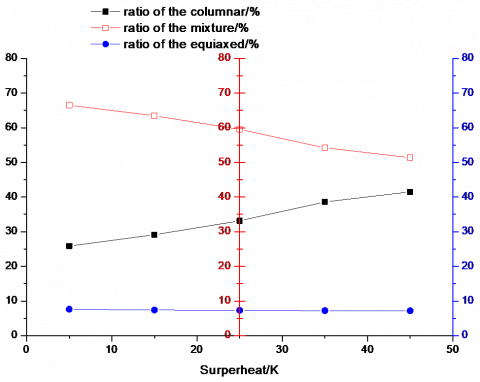Figure 5. Effect of surperheat on the ratio of the columnar, equiaxed and mixture

The surperheat has little effect on the ratio of the equiaxed area when the casting speed is 0.030m/s. But the ratio of the mixture area becomes smaller with the surperheat increasing, and the ratio of the columnar area becomes larger with the surperheat increasing. The $d \varphi_{i} / d \Delta T_{s}$ is approximately constant, and 0.42%/K for columnar area, -0.41%/K for mixture area and -0.01%/K for equiaxed area.

3.2 Effects of casting speed on the ratio of the columnar, equiaxed and mixture

The results of solidification structure with different velocity calculated by the CAFE model  are shown in Figure 6, and the predictions by the CET model are shown in Figure 7.

## 6.png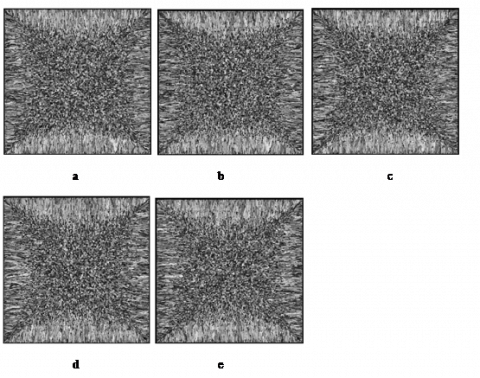Figure 6. Calculated by CAFE model  with $\Delta T_{s}$=25K a) $U_{\text {cast}}$=0.043m/s b) $U_{\text {cast}}$=0.037m/s c) $U_{\text {cast}}$=0.030m/s d) $U_{\text {cast}}$=0.023m/s e) $U_{\text {cast}}$=0.017m/s

## 7.png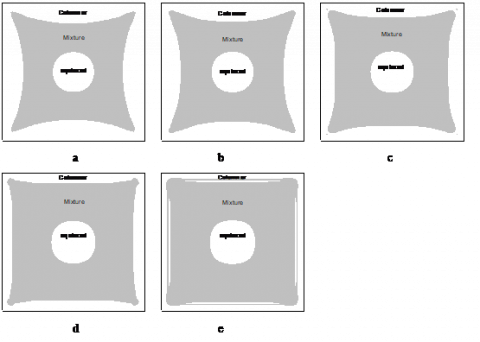Figure 7. Calculated by CET model with $\Delta T_{s}$=25K a) $U_{\text {cast}}$=0.043m/s b) $U_{\text {cast}}$=0.037m/s c) $U_{\text {cast}}$=0.030m/s d) $U_{\text {cast}}$=0.023m/s e) $U_{\text {cast}}$=0.017m/s

The comparison between Figures 6 and 7 shows that the calculation of this work can instead be used with the CAFE model to study the effect of casting speed on the ratio of the columnar, equiaxed and mixture. As the casting speed increases, the mixture area becomes larger and the equiaxed area becomes smaller, but the columnar area has little change which is shown in sections a~e of Figure 7. But this phenomenon does not show in Figure 6. The ratios of each area which are statistically analyzed from sections a~e of Figure 7 are shown in Figure 8.

## 8.pngFigure 8. Effect of casting speed on the ratio of the columnar, equiaxed and mixture

The casting speed has little effect on the ratio of the equiaxed area, which when the surperheat is 25K. But as the casting speed increases, the ratio of the mixture area becomes smaller and the ratio of the columnar area becomes larger. The $d \varphi_{i} / d U_{c a s t}$ is approximately constant at 910.70%.s.m-1 for columnar area, -851.1%.s.m-1 for mixture area, -59.57%.s.m-1 for equiaxed area.

3.3 Effects of zone cooling rate on the ratio of the columnar, equiaxed and mixture

## 9a.png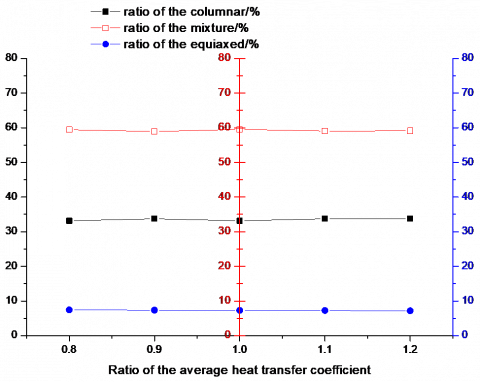a)

## 9b.png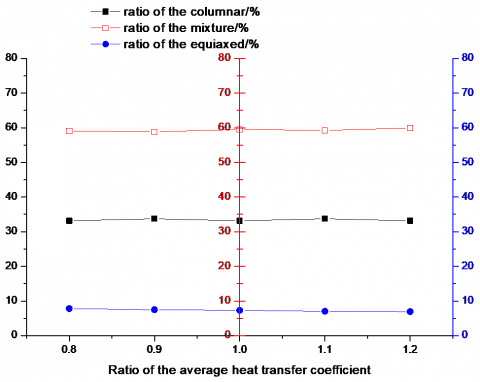b)

## 9c.png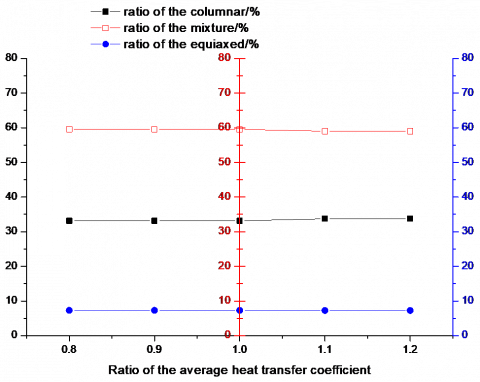c)

Figure 9. Effect of zone cooling rate on the ratio of the columnar, equiaxed and mixture, a) Zone 1, b) Zone 2, c) Zone 3

The effects of zone cooling rate on the ratio of the columnar, equiaxed and mixture are shown in sections a, b and c of Figure 9. The average heat transfer coefficient ratios have little effect on the ratios of the columnar, equiaxed and mixture for there is little change in the ratio of the columnar, equiaxed and mixture when the ratio of the average heat transfer coefficient of Zones 1, 2, and 3 changes.

4. Conclusions

This work studies the factors influencing the columnar-equiaxed transition in the 0.17m$\times$0.17m billet casting process. Effects of zone surperheat, casting speed and cooling rate on the ratio of the columnar, equiaxed and mixture are given, and the following conclusions can be established.

a) The main two influencing factors on the columnar-equiaxed transition in the 0.17m$\times$0.17m billet casting process are surperheat and cooling rate after considering that the cooling rate decreases with the casting speed increasing.

b) The surperheat has little effect on the ratio of the equiaxed area, but as the surperheat increases the ratio of the mixture area becomes smaller, and the ratio of the columnar area becomes larger. The $d \varphi / d \Delta T_{s}$ is approximately constant at 0.42%/K for columnar area, -0.41%/K for mixture area, -0.01%/K for equiaxed area.

c) The casting speed has little effect on the ratio of the equiaxed area, but as the casting speed increases the ratio of the mixture area becomes smaller, and the ratio of the columnar area becomes larger. The $d \varphi_{i} / d U_{c a s t}$ is approximately constant at 910.70%.s.m-1 for columnar area, -851.12%.s.m-1  for mixture area, -59.57%.s.m-1 for equiaxed area.

d) The average heat transfer coefficient ratios have little effect on the ratios of the columnar, equiaxed and mixture.

Acknowledgment

The authors gratefully express their appreciation to National Natural Science Fund of China (51174024, U1360201) for sponsoring this work.

References

1. J. D. Hunt, Steady State Columnar and Equiaxed Growth of Dendrites and Eutectic, Materials Science and Engineering, vol.65, pp.75-83, 1984. DOI: 10.1016/0025-5416(84)90201-5.

2. M. A. Martorano, C. Beckermann and C. A. Gandin, A Solutal Interaction Mechanism for the Columnar-to- Equiaxed Transition in Alloy Solidification, Metallurgical and Materials Transactions A-Physical Metallurgy and Materials Science, vol.34, pp.1657- 1674, 2003. DOI: 10.1007/s11661-003-0311-x.

3. W. Kurz, B. Giovanola and R. Trivedi, Theory of Microstructural Development during Rapid Solidification, Acta Metallurgica et Materialia, vol.34, pp.823-830, 1986. DOI: 10.1016/0001-6160(86)90056- 8.

4. W. Kurz, C. Bezencon and M. Gäumann, Columnar to Equiaxed Transition in Solidification Processing, Science and Technology of Advanced Materials, vol.2, pp.185-191, 2001. DOI: 10.1016/S1468- 6996(01)00047-X.

5. C. Gandin and M. Rappaz, A Coupled Finite Element- Cellular Automaton Model for the Prediction of Dendritic Grain Structures in Solidification Processes, Acta Metallurgica et Materialia, vol.42, pp.2233-2246, 1994. DOI: 10.1016/0956-7151(94)90302-6.

6. M. Rappaz and C. Gandin, Probabilistic Modelling of Microstructure Formation in Solidification Processes, Acta Metallurgica et Materialia, vol.41, pp.345-360, 1993. DOI: 10.1016/0956-7151(93)90065-Z.

7. L. Nastac, Numerical Modeling of Solidification Morphologies and Segregation Patterns in Cast Dendritic Alloys, Acta Materialia, vol.47, pp.4253- 4262, 1999. DOI: 10.1016/S1359-6454(99)00325-0.

8. C. Gandin and M. Rappaz, A Coupled Finite Element- Cellular Automaton Model for the Prediction of Dendritic Grain Structures in Solidification Processes, Acta Metallurgica et Materialia, vol.42, pp.2233-2246, 1994. DOI: 10.1016/0956-7151(94)90302-6.

9. M. Rappaz and C. Gandin, Probabilistic Modelling of Microstructure Formation in Solidification Processes, Acta Metallurgica et Materialia, vol.41, pp.345-360, 1993. DOI: 10.1016/0956-7151(93)90065-Z.

10. L. Nastac, Numerical Modeling of Solidification Morphologies and Segregation Patterns in Cast Dendritic Alloys, Acta Materialia, vol.47, pp.4253- 4262, 1999. DOI: 10.1016/S1359-6454(99)00325-0.

11. L. Nastac and D. M. Stefanescu, Stochastic Modelling of Microstructure Formation in Solidification Processes, Modelling and Simulation in Materials Science and Engineering, vol.5, pp.391, 1997. DOI: 10.1088/0965- 0393/5/4/008.

12. H. Zibing, Compactness Degree of Solidification Structure and Characteristics and Quantitative Model of Macrosegregation in Continuous Casting Billet of High- Carbon Steel, Doctor, School of Metallurgical and Ecological Engineering, University of Science and Technology Beijing, Beijing, 2012.

13. Y. Meng and B. G. Thomas, Heat-transfer and Solidification Model of Continuous Slab Casting: CON1D, Metallurgical And Materials Transactions B- Process Metallurgy And Materials Processing Science, vol.34, pp.685-705, 2003. DOI: 10.1007/s11663-003- 0040-y.

14. R. Davies, N. Blake and P. Campbell, Solidification Modelling--an Aid to Continuous Casting, 4 th International Conference Continuous Casting, vol.2, pp.645-654, 1988.